Journal of Integer Sequences, Vol. 17 (2014), Article 14.8.6

## On the Powerful and Squarefree Parts of an Integer

### Maurice-Étienne Cloutier, Jean-Marie De Koninck, and Nicolas Doyon Département de mathématiques et de statistique Université Laval Québec G1V 0A6 Canada

Abstract:

Any integer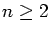can be written in a unique way as the product of its powerful part and its squarefree part, that is, as n=mr where m is a powerful number and r a squarefree number, with gcd(m,r)=1. We denote these two parts of an integer n by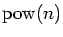and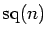respectively, setting for convenience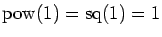. We first examine the behavior of the counting functions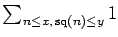and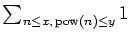. Letting P(n) stand for the largest prime factor of n, we then provide asymptotic values of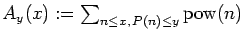and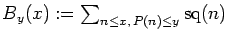when y=x1/u withfixed. We also examine the size of Ay(x) and By(x) when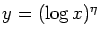for some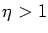. Finally, we prove that Ay(x) will coincide with By(x) in the sense that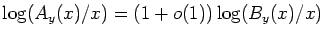as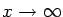if we choose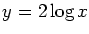.

Full version:  pdf,    dvi,    ps,    latex

Received July 8 2014; revised version received July 31 2014. Published in Journal of Integer Sequences, August 5 2014.